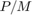# FIR Decimation Using Single-Rate Processing

In this example, the FIR Decimation block decimates the input signal by decreasing its frame size while maintaining the sample rate.

Open the model. The input to the FIR Decimation block is a single-channel fixed-size input with a frame size of 64. In the block dialog box, set the Decimation factor parameter to 4, Input processing to `Columns as channels (frame based)`, Rate options to `Enforce single-rate processing`, and clear the Allow arbitrary frame length for fixed-size input signals parameter.

Run the Model. The block operates in the single-rate processing mode and does not allow arbitrary input frame length. The output frame size of the block istimes the input frame size of the block, where M is the decimation factor. With a decimation factor of 4, the output has a frame size of 16. As shown in the model, the input and the output of the FIR Decimation block have the same sample rate but different frame sizes.If you select the Allow arbitrary frame length for fixed-size input signals parameter, the input frame length P does not have to be a multiple of the decimation factor. In this case, the output frame length has an upper bound of `ceil`().